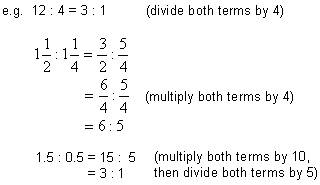# Ratios Math Review

math tutorials > ratios tutorial

## What is a ratio?

A ratio is a method of comparing 2 or more quantities that are measured in the same units.

A ratio is usually written with the quantities separated by a colon e.g. 4 : 5, but the 2 quantities can also be compared by writing them as a fraction.

## Simpifying Ratios

Ratios are simplified similarly to fractions.## Note:

• Ratios are normally written as whole numbers.  Where possible they should not   include decimals, fractions or percentages.
• Ratios do not have units.  Quantities with units must first be converted to the same unit, then simplified as a ratio.

e.g.  5cm : 20mm = 50mm : 20mm = 5 : 2

## Ratio problems for you to try.

A.  Simplify:
1)  15 : 3      2)  6min : 1hr      3)  15 : 20

B.  Find x:
1)  12 : x = 6 : 2      2)  x : 3 = 15 : 9

Solutions:

A.  1)  5 : 1    2)  1 : 10    3)  3 : 4
B.  1)  x = 4    2)  x = 5